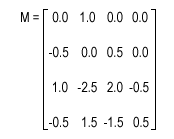Prev: Ray-Triangle Intersection Next: Vector Length

The Catmull-Rom cubic spline takes a set of key frame values to describe a smooth piecewise cubic curve that passes through all the keys.

In order to use this routine we need four key frame values. So we have v0,v1,v2, and v3, plus a parameter that specifies a key, x between v1 and v2 to interpolate. The return value, f(x) is the value associated with the key x.

`   	f(x) = [1, x, x^2, x^3] * M * [v0, v1, v2, v3]`

where M is defined as:The image bellow shows an example of a curve between v1 and v2. The curve was obtained calling the function bellow varying x between 0.0 and 1.0.

```	for (x=0;x<=1;x+=0.01)
printf("%f\n",catmullRomSpline(x,v0,v1,v2,v3));```The following C code performs the required operation. The return value is the value for the parameter key x. The remaining parameters are the four values as mentione above. Note that x is assumed to be between v1 and v2 . The value for x must be between 0.0 and 1.0. If x = 0.0 then the return value is v1. For x = 1.0, the return value is v2.

```/* Coefficients for Matrix M */
#define M11	 0.0
#define M12	 1.0
#define M13	 0.0
#define M14	 0.0
#define M21	-0.5
#define M22	 0.0
#define M23	 0.5
#define M24	 0.0
#define M31	 1.0
#define M32	-2.5
#define M33	 2.0
#define M34	-0.5
#define M41	-0.5
#define M42	 1.5
#define M43	-1.5
#define M44	 0.5

double catmullRomSpline(float x, float v0,float v1,
float v2,float v3) {

double c1,c2,c3,c4;

c1 =  	      M12*v1;
c2 = M21*v0          + M23*v2;
c3 = M31*v0 + M32*v1 + M33*v2 + M34*v3;
c4 = M41*v0 + M42*v1 + M43*v2 + M44*v3;

return(((c4*x + c3)*x +c2)*x + c1);
}```

 Prev: Ray-Triangle Intersection Next: Vector Length

### 3 Responses to “Catmull-Rom Spline”

1.Are you able to explain the general rule for working with more than four points? Might sound stupid, but just clarifying, four is the minimum right but you could increase this number!?

•Hi,

The first four points give you the first curve segment. Applying the same algorithm to points v1 to v4 (also a set of four points you get the second segment of the curve, this time between v2 and v3.

Hope this helps,

Antonio

2.If we want the key frames on a specific time, v0..1 must be 2D points, with (time, value), am I right?

If so how can the Catmull-Rom spline be calculated?

This site uses Akismet to reduce spam. Learn how your comment data is processed.